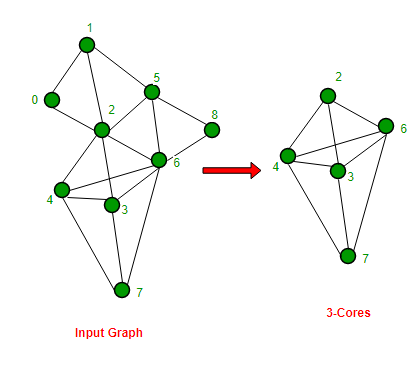Find k-cores of an undirected graph

• Difficulty Level : Hard
• Last Updated : 18 Jun, 2021

Given a graph G and an integer K, K-cores of the graph are connected components that are left after all vertices of degree less than k have been removed (Source wiki)

Example:

Input : Adjacency list representation of graph shown
on left side of below diagram
Output: K-Cores :
 -> 3 -> 4 -> 6
 -> 2 -> 4 -> 6 -> 7
 -> 2 -> 3 -> 6 -> 7
 -> 2 -> 3 -> 4 -> 7
 -> 3 -> 4 -> 6We strongly recommend you to minimize your browser and try this yourself first.
The standard algorithm to find a k-core graph is to remove all the vertices that have degree less than- ‘K’ from the input graph. We must be careful that removing a vertex reduces the degree of all the vertices adjacent to it, hence the degree of adjacent vertices can also drop below-‘K’. And thus, we may have to remove those vertices also. This process may/may not go until there are no vertices left in the graph.

To implement above algorithm, we do a modified DFS on the input graph and delete all the vertices having degree less than ‘K’, then update degrees of all the adjacent vertices, and if their degree falls below ‘K’ we will delete them too.

Below is implementation of above idea. Note that the below program only prints vertices of k cores, but it can be easily extended to print the complete k cores as we have modified adjacency list.

C#

Output :

K-Cores :

 -> 3 -> 4 -> 6
 -> 2 -> 4 -> 6 -> 7
 -> 2 -> 3 -> 6 -> 7
 -> 2 -> 3 -> 4 -> 7
 -> 3 -> 4 -> 6

K-Cores :

Time complexity of the above solution is O(V + E) where V is number of vertices and E is number of edges.

Related Concepts :
Degeneracy : Degeneracy of a graph is the largest value k such that the graph has a k-core. For example, the above shown graph has a 3-Cores and doesn’t have 4 or higher cores. Therefore, above graph is 3-degenerate.
Degeneracy of a graph is used to measure how sparse graph is.

Reference :
https://en.wikipedia.org/wiki/Degeneracy_%28graph_theory%29